• （16）VHDL实现半加器与全加器
2021-11-10 16:21:37

## 1.1 目录

1）目录

2）FPGA简介

3）VHDL简介

4）VHDL实现半加器与全加器

5）结语

## 1.2 FPGA简介

FPGA（Field Programmable Gate Array）是在PAL、GAL等可编程器件的基础上进一步发展的产物。它是作为专用集成电路（ASIC）领域中的一种半定制电路而出现的，既解决了定制电路的不足，又克服了原有可编程器件门电路数有限的缺点。

FPGA设计不是简单的芯片研究，主要是利用 FPGA 的模式进行其他行业产品的设计。 与 ASIC 不同，FPGA在通信行业的应用比较广泛。通过对全球FPGA产品市场以及相关供应商的分析，结合当前我国的实际情况以及国内领先的FPGA产品可以发现相关技术在未来的发展方向，对我国科技水平的全面提高具有非常重要的推动作用。

与传统模式的芯片设计进行对比，FPGA 芯片并非单纯局限于研究以及设计芯片，而是针对较多领域产品都能借助特定芯片模型予以优化设计。从芯片器件的角度讲，FPGA 本身构成 了半定制电路中的典型集成电路，其中含有数字管理模块、内嵌式单元、输出单元以及输入单元等。在此基础上，关于FPGA芯片有必要全面着眼于综合性的芯片优化设计，通过改进当前的芯片设计来增设全新的芯片功能，据此实现了芯片整体构造的简化与性能提升。

以硬件描述语言（Verilog或VHDL）所完成的电路设计，可以经过简单的综合与布局，快速的烧录至 FPGA 上进行测试，是现代 IC设计验证的技术主流。这些可编辑元件可以被用来实现一些基本的逻辑门电路（比如AND、OR、XOR、NOT单片机 嵌入式硬件 开发语言
更多相关内容
• 目的：用VHDL文本输入法设计并实现一个一位半加器。 程序（使用quartus II软件进行编写） library ieee; use ieee.std_logic_1164.all; entity add1_half is port( Ai,Bi :in std_logic; So,Co :out ...

目的：用VHDL文本输入法设计并实现一个一位半加器。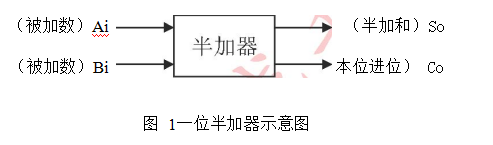程序（使用quartus II软件进行编写）

library ieee;
use ieee.std_logic_1164.all;

port(
Ai,Bi		:in std_logic;
So,Co		:out std_logic		--So半加和,Co进位输出
);

begin
process(Ai,Bi)
begin
So <= Ai xor Bi;
Co <= Ai and Bi;
end process;
end behave;


仿真波形图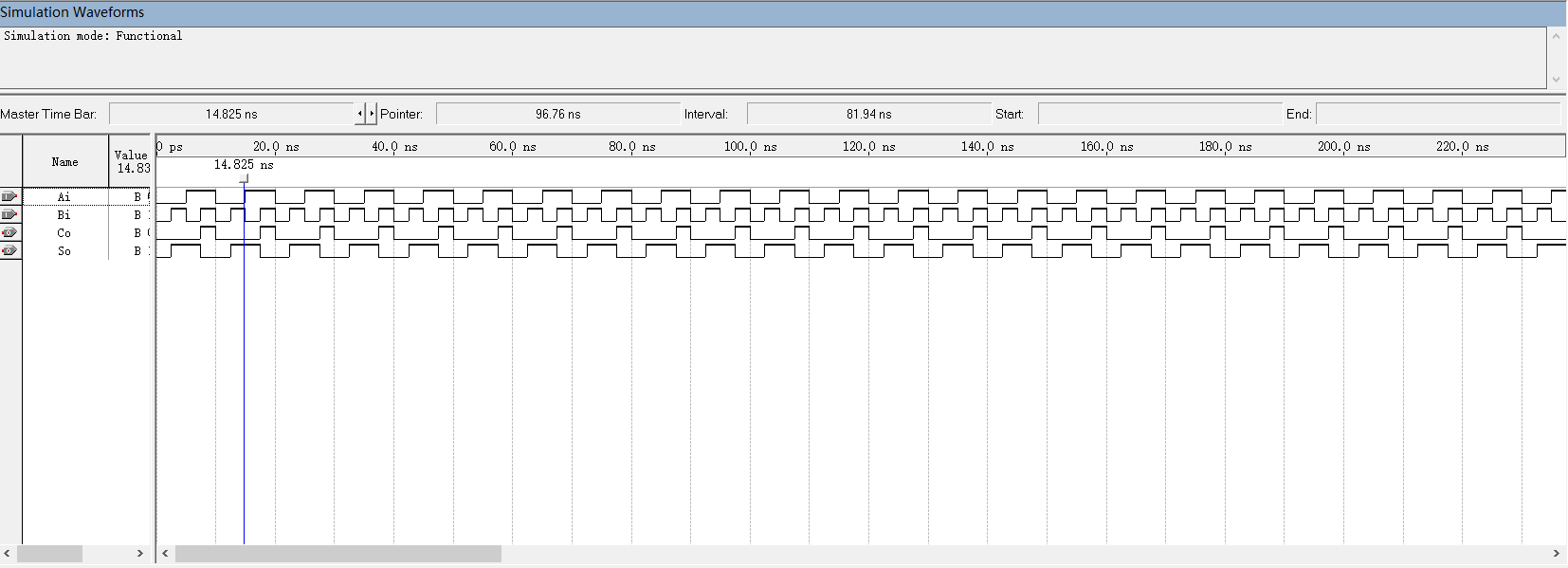展开全文fpga开发
• 利用VHDL语言能够设计电子系统，本文档就是用VHDL描述设计半加器，可以让大家初步认识VHDL设计的基本流程以及与其他程序设计语言的区别。
• 4.VHDL语言 library ieee; use ieee.std_logic_1164.all; entity h_adder is port (a,b:in STD_LOGIC; co,so:out STD_LOGIC); end h_adder; architecture fh of h_adder is signal sel:STD_LOGIC_VECTOR(1 ...

1.真值表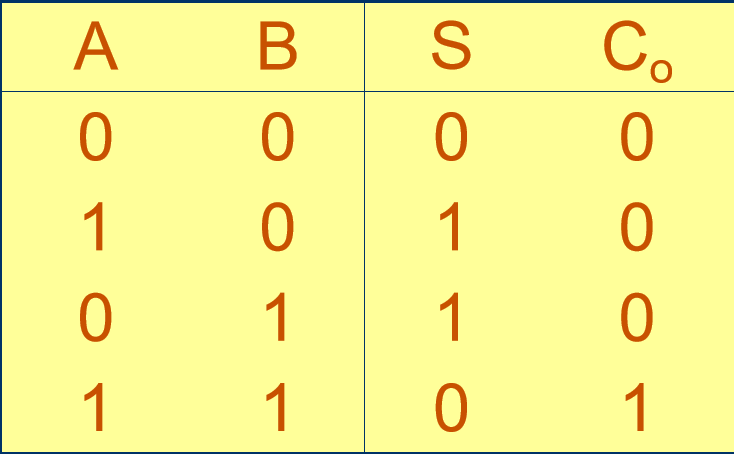2.逻辑电路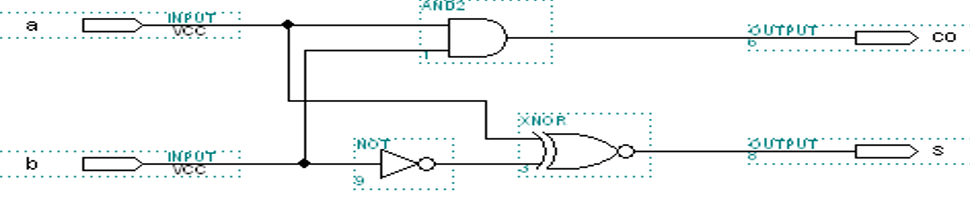3.端口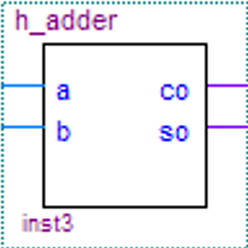4.VHDL语言
4.1.表达1：

library ieee;
use ieee.std_logic_1164.all;

port (a,b:in STD_LOGIC;
co,so:out STD_LOGIC);

signal sel:STD_LOGIC_VECTOR(1 downto 0);
begin
sel <= a&b;
process(sel)
begin
case sel is
when "00"=>so<='0';co<='0';
when "01"=>so<='1';co<='0';
when "10"=>so<='1';co<='0';
when "11"=>so<='0';co<='1';
when others=>null;
end case;
end process;
end fh;


4.2表达2：

library ieee;
use ieee.std_logic_1164.all;

port (a,b:in STD_LOGIC;
co,so:out STD_LOGIC);

begin
so <= not(a xor (not b));
co <= a and b;
end fh;

展开全文• 选了FPGA的课记录一下怎么搭建工程免得忘了 1、先创建工程 然后记得选ISim 要不然等会仿真的...然后 VHDL Module 接着输入下面的信息 3、编写VHDL代码 输入以下代码 library IEEE; use IEEE.STD_LOGI...

# 1、先创建工程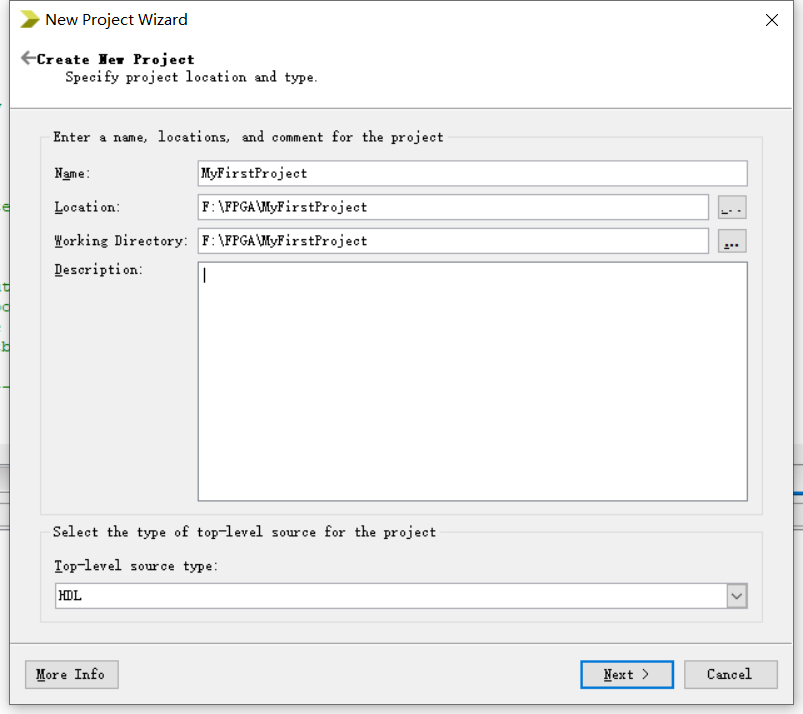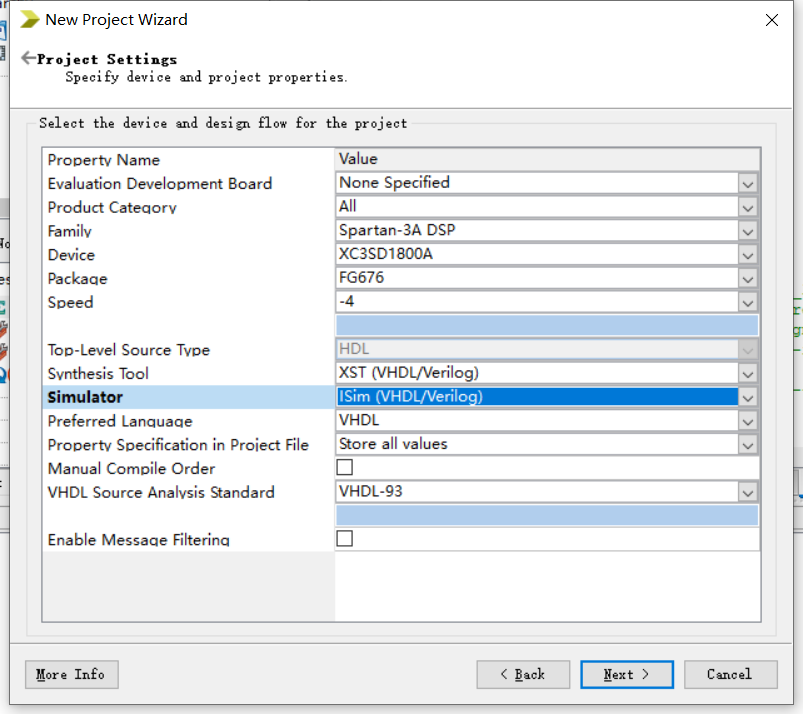然后记得选ISim 要不然等会仿真的时候会让你使用modelsim的仿真器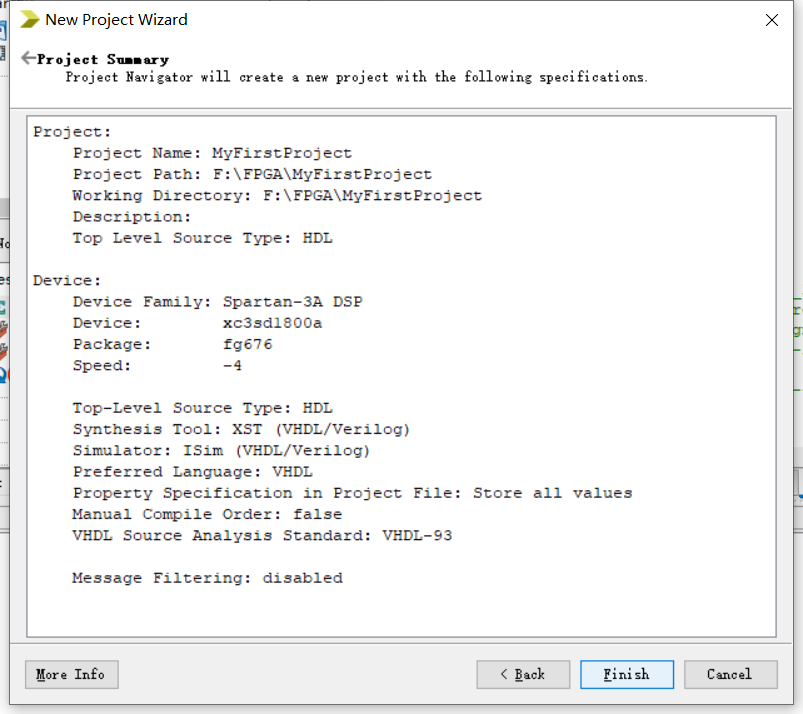完成创建 Finish

# 2、添加模块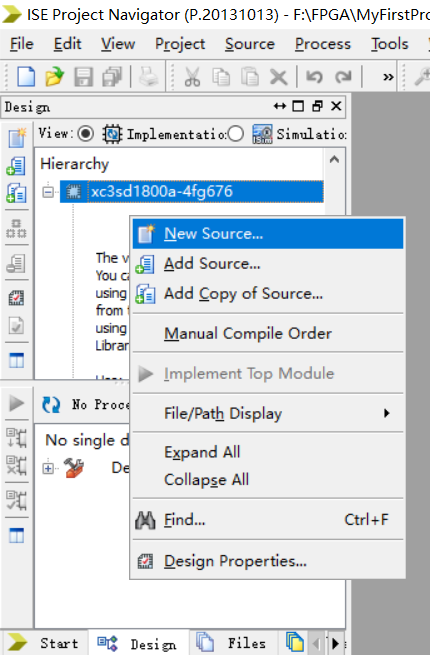选择new source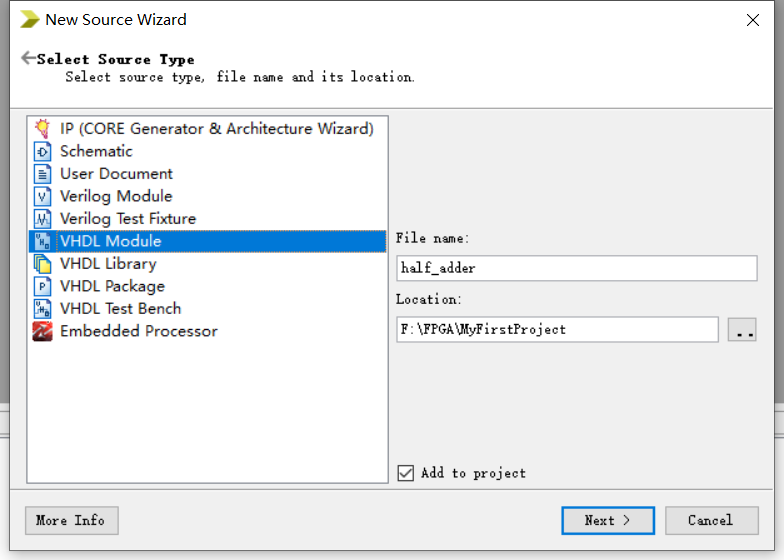然后 VHDL Module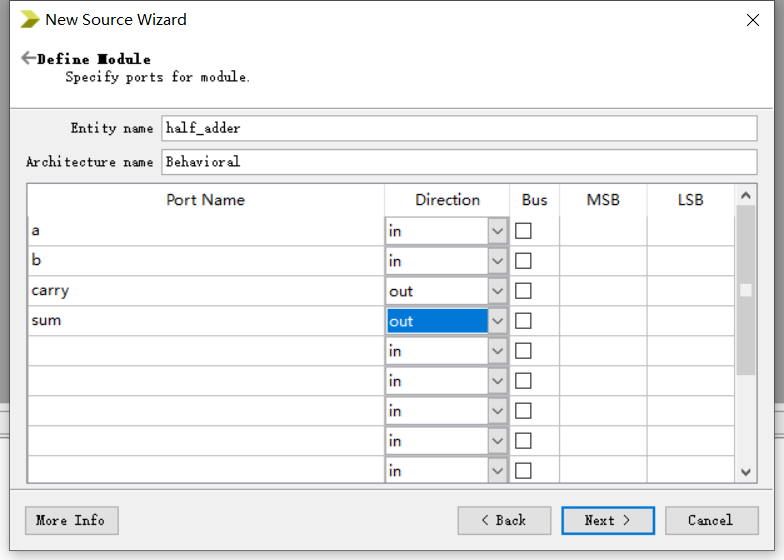接着输入下面的信息

# 3、编写VHDL代码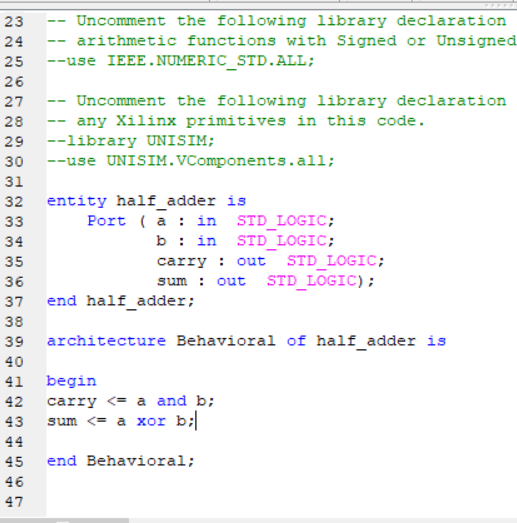输入以下代码

library IEEE;
use IEEE.STD_LOGIC_1164.ALL;

-- Uncomment the following library declaration if using
-- arithmetic functions with Signed or Unsigned values
--use IEEE.NUMERIC_STD.ALL;

-- Uncomment the following library declaration if instantiating
-- any Xilinx primitives in this code.
--library UNISIM;
--use UNISIM.VComponents.all;

Port ( a : in  STD_LOGIC;
b : in  STD_LOGIC;
carry : out  STD_LOGIC;
sum : out  STD_LOGIC);

begin
carry <= a and b;
sum <= a xor b;

end Behavioral;



检查语法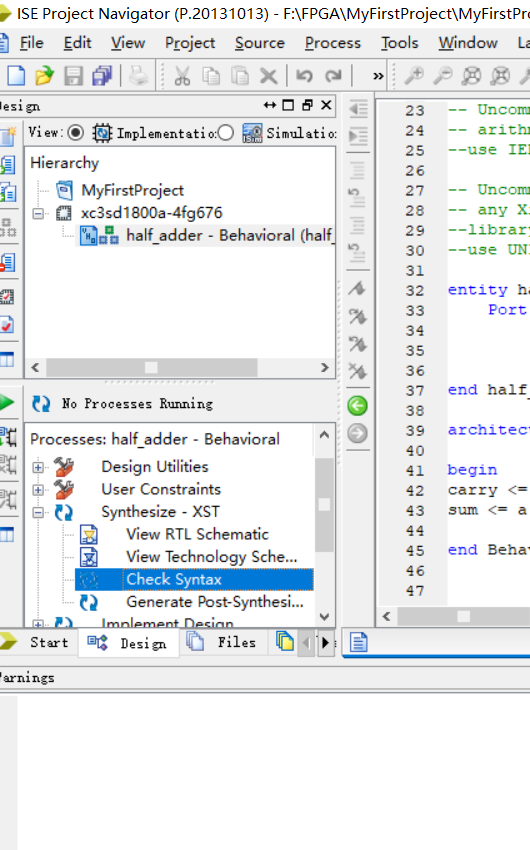检查语法  check syntax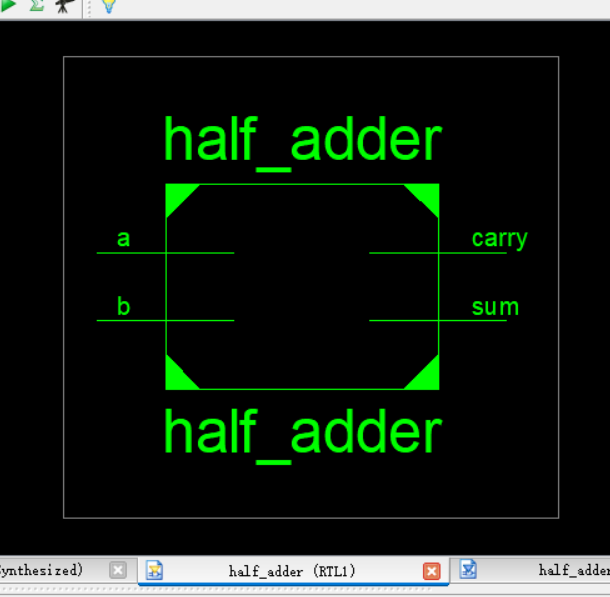view RTL Schematic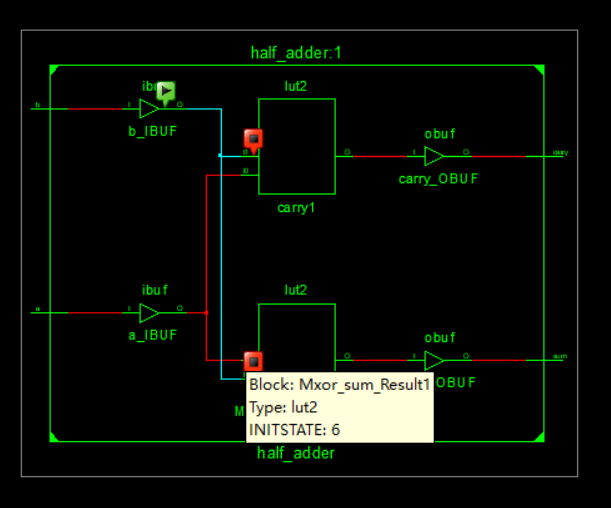双击上面那个 RTL Schematic   可以查看里面的原件    确认没问题后就 开始仿真

# 4、仿真

design 那里选择 simulation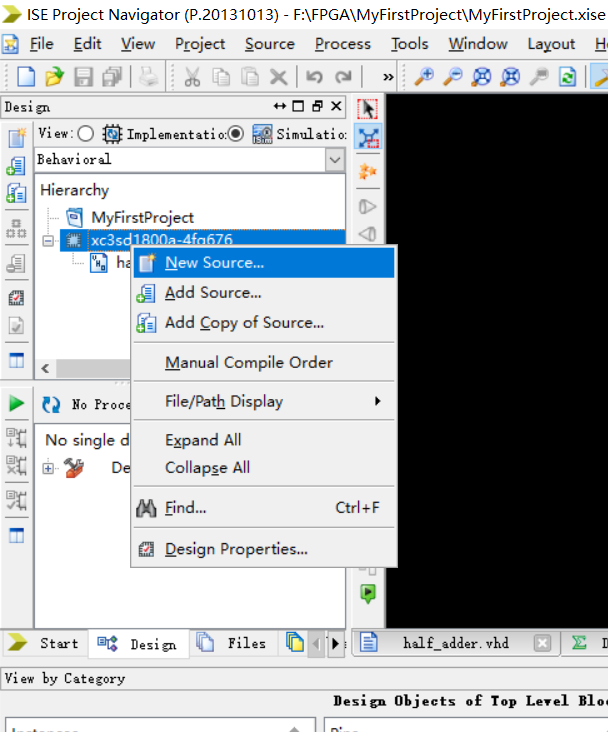New Source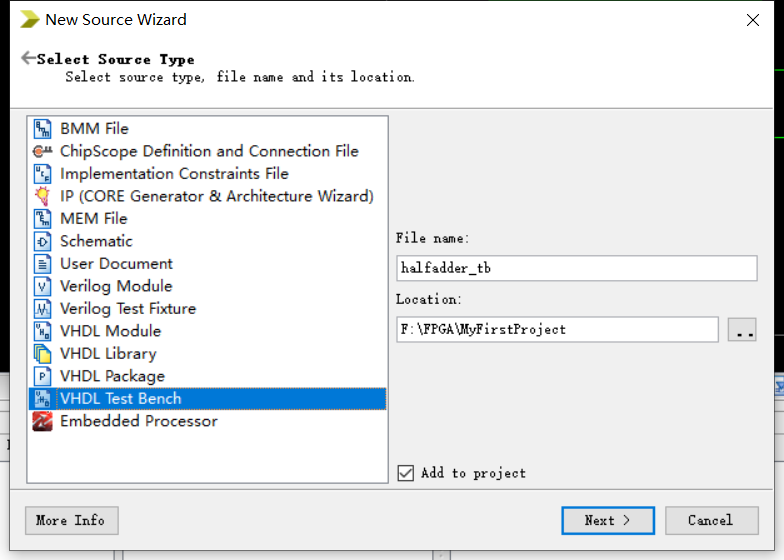创建teset bench

然后写仿真的test bench

LIBRARY ieee;
USE ieee.std_logic_1164.ALL;

-- Uncomment the following library declaration if using
-- arithmetic functions with Signed or Unsigned values
--USE ieee.numeric_std.ALL;

ENTITY test IS
END test;

ARCHITECTURE behavior OF test IS

-- Component Declaration for the Unit Under Test (UUT)

PORT(
a : IN  std_logic;
b : IN  std_logic;
carry : OUT  std_logic;
sum : OUT  std_logic
);
END COMPONENT;

--Inputs
signal a : std_logic := '0';
signal b : std_logic := '0';

--Outputs
signal carry : std_logic;
signal sum : std_logic;
-- No clocks detected in port list. Replace <clock> below with
-- appropriate port name

BEGIN

-- Instantiate the Unit Under Test (UUT)
a => a,
b => b,
carry => carry,
sum => sum
);

-- Clock process definitions

-- Stimulus process
stim_proc: process
begin
-- hold reset state for 100 ns.
wait for 100 ns;
a <= '0';
b <= '0';
wait for 100 ns;
a <= '0';
b <= '1';
wait for 100 ns;
a <= '1';
b <= '0';
wait for 100 ns;
a <= '1';
b <= '1';
wait for 100 ns;

-- insert stimulus here

wait;
end process;

END;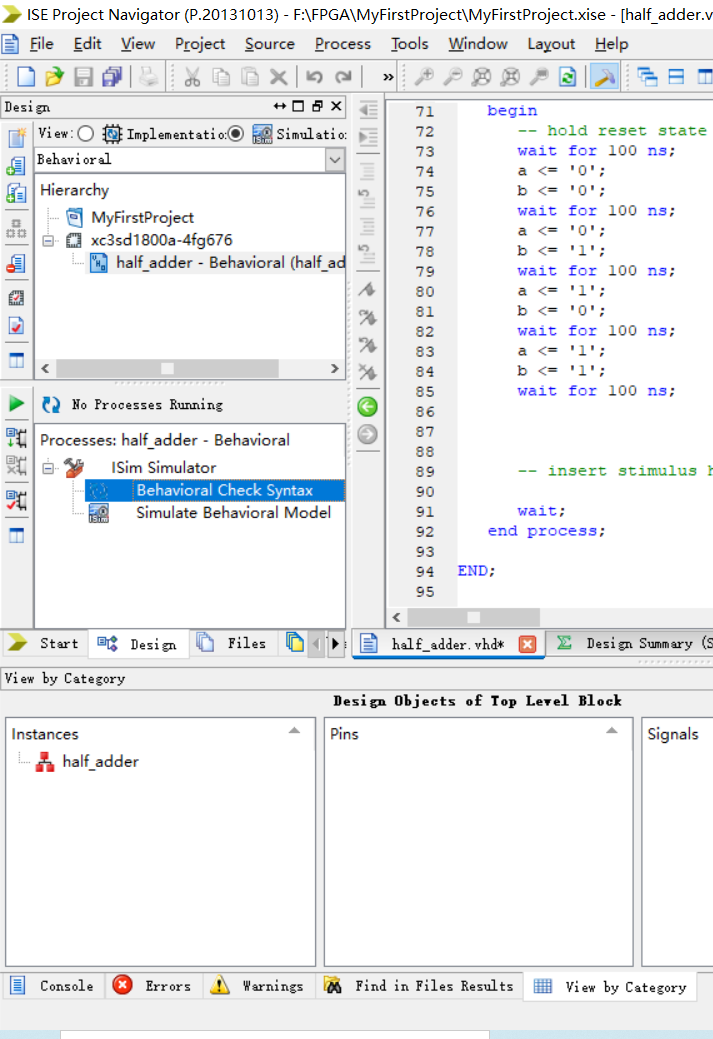确认语法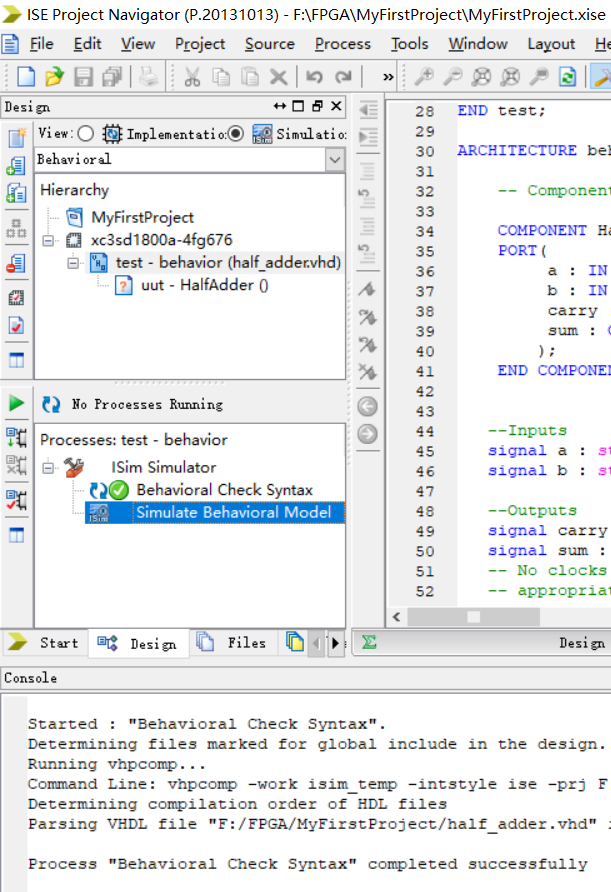开始仿真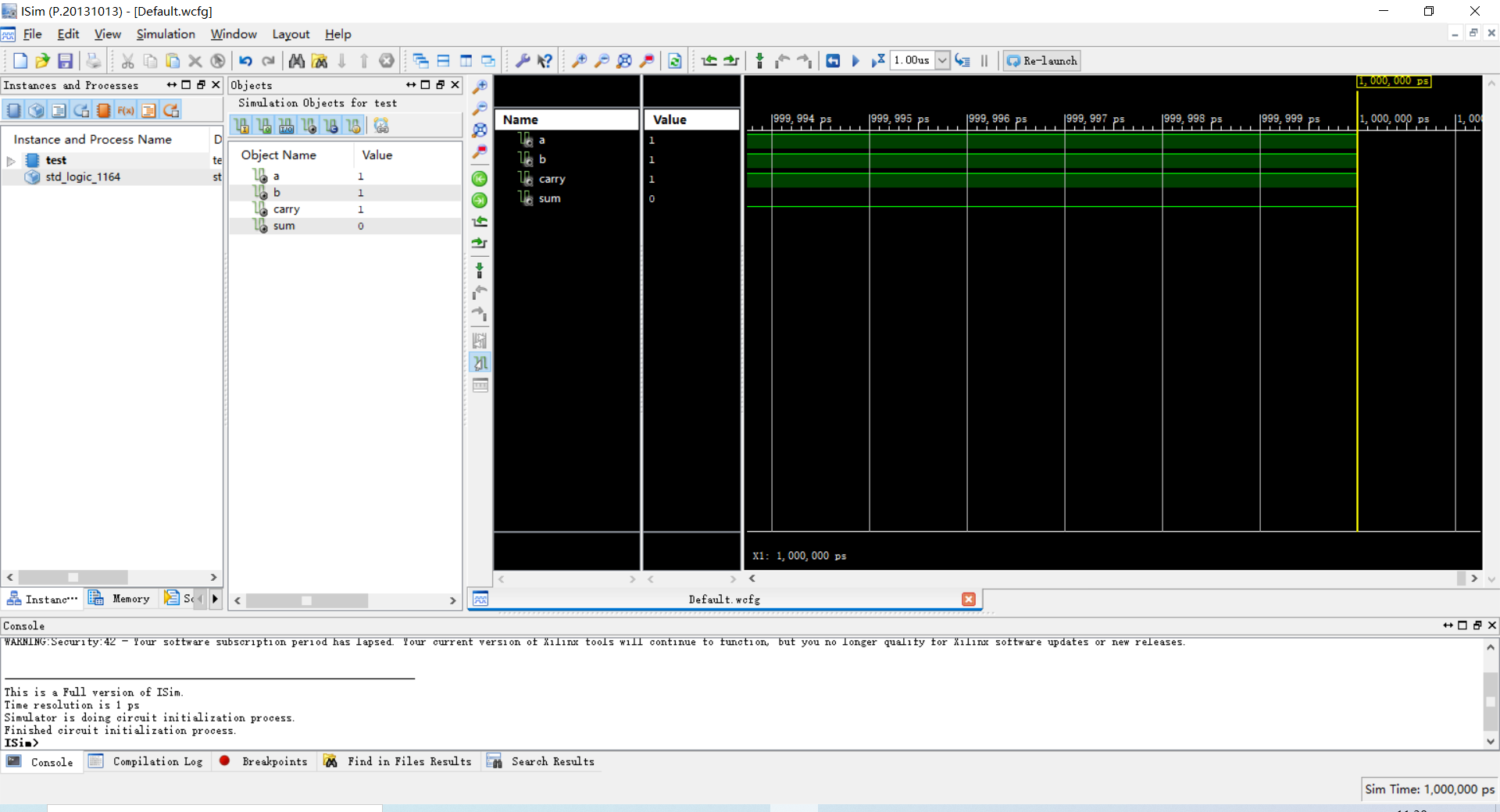出来如下界面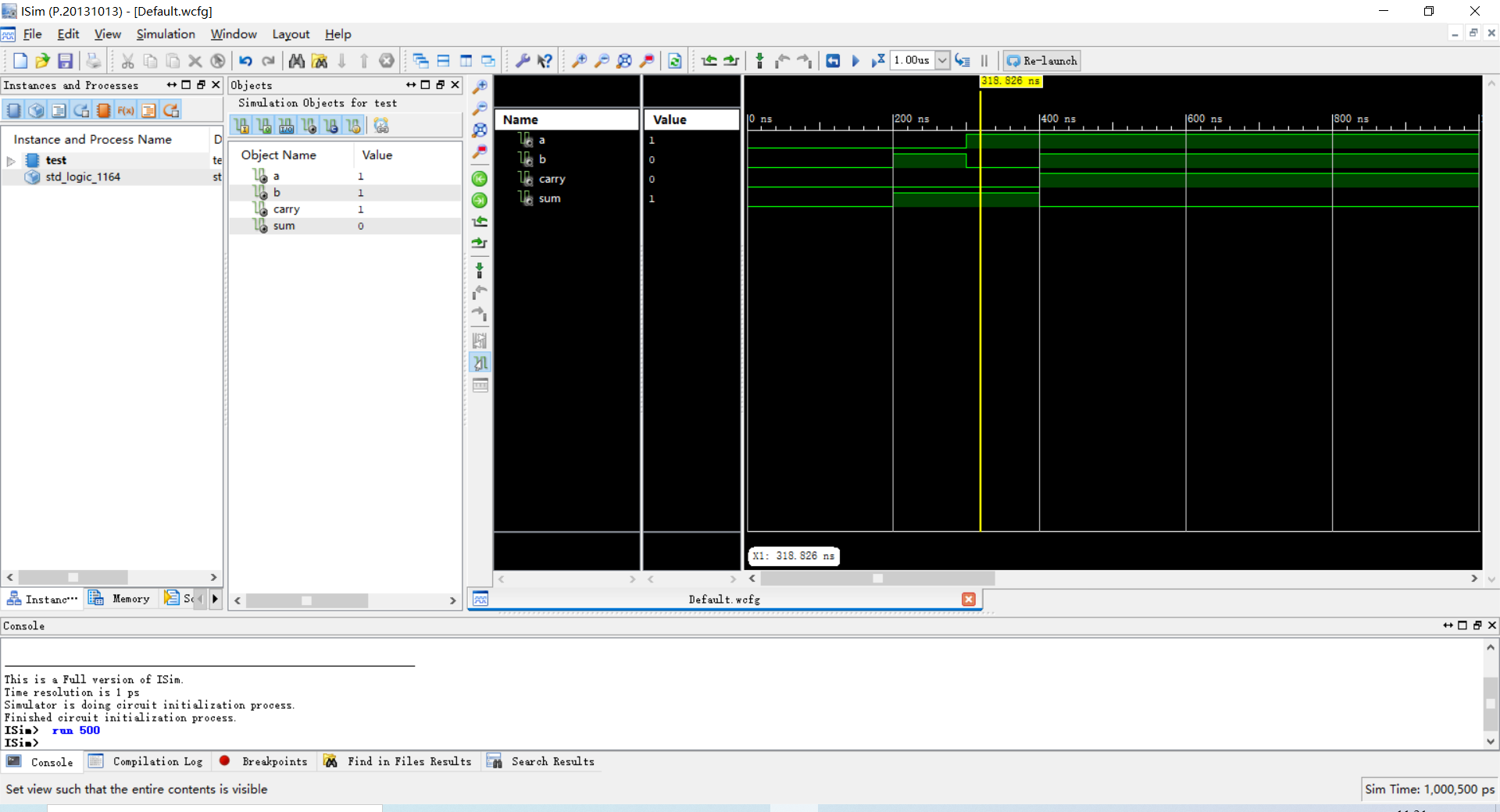在ISim 下面输入 run 500 出现如下波形  确认仿真结果以后  仿真结束。

展开全文• 本文档是我在接触Active-HDL这个上上机工具1天内写出来的，意在记录从VHDL源程序、测试平台代码到仿真输出方波的过程、注意事项，难免有些简单、肤浅。 请高手勿拍砖，直接忽视这篇小儿科文档； 也希望热心的前辈...测试
• 1.半加器   2.   3.或门嵌入式
• 一百个例子的VHDL语言例代码，含有2选1多路选择器程序，n输入与非门的实体描述等等
• // 半加器 `timescale 1ns/10ps module h_adder( a, b, so, co ); input a; input b; output so; // 进位，异或 so = a^b; output co; // 本位，与，co = a&b; assign so = ...verilog
• 用门电路实现两个二进数相加并求出和的组合线路，称为一个全加器。
• vhdl 语言用结构描述法实现半加器，包括底层实体和顶层实体
• 一. 实验说明 使用软件：Quartus II 5.0 实验要求说明：设计一个一位全加器（full adder） 1.引脚功能表 逻辑说明：XOR 为异或 ，...2. VHDL 实现 use ieee.std_logic_1164.all; entity full_adder1 is p...
• VHDL主要用于描述数字系统的结构，行为，功能和接口。除了含有许多具有硬件特征的语句外，VHDL的语言形式和描述风格与句法是十分类似于一般的计算机高级语言。用结构方法设计一个半加器。两个一位二进制数Ai 和Bi...
• VHDL 仿真的 半加器 Quartus II开发环境 参考文献 《VHDL电路设计》P36
• EDA实验和毕业设计的同学应该用的到！！ 常见的都有
• 1 位全加器可以用两个半加器及一个或门连接而成，半加器原理图的设计方法很多，我 们用一个与门、一个非门和同或门（xnor 为同或符合,相同为 1,不同为 0）来实现。先设计 底层文件：半加器,再设计顶层文件全加器。...fpga开发
• 基于quartus II实现的全加器 内含实现全加器的VHDL代码、逻辑图（BDF）以及激励波形文件（VWF）。
• 该资源是基于VHDL语言在Quartus平台上实现全加器的设计 采用顶层和底层的设计 底层的半加器VHDL或者原理图来实现
• 基于VHDL语言的全加器的设计 全加器可以由两个半加器和一...把全加器、半加器、或门的vhdl文件都要包含到工程中； 在全加器文件中声明半加器、或门为元件； 然后例化三个元件：两个半加器和一个或门。 软件说明：Mo...全加器
• ## VHDL加法器

千次阅读 2017-10-02 16:03:43
教材：VHDL硬件描述语言与数字逻辑电路设计（第三版） 软件：Quartus II   熟悉软件基本操作，设计简单的加法器，并进行仿真实验。 library ieee; use ieee.std_logic_1164.all; entity my_and is port ( a,b ...
• 数字逻辑与系统设计，转为用VHDL语言学习者，上机实验者，不能很好的用结构描述半加器所打造。
• 在乘法器电路中，乘数中的每一位都要和被乘数的每一位相与，并产生其相应的乘积位。这些局部乘积要馈入到全加器的阵列中（合适的时候也可以用半加器），同时加法器向左移位并表示出乘法结果。
• vhdl实验中常见的几个实验，包括数据选择器，加法器，d触发器，音乐演奏
• 1位全加器接口有：A被加数输入端，为加数输入端，C进位输入端，CO进位输出端，S和数输出端。1位全加器表达式如下：S=A xor B xor C, CO=(AB)+(AC)...本文用VHDL语言的GENERATE语句用四个一位全加器实现一个四位全加器。
• vhdl常用的语法无非就 用在进程下边： 1.if-else-elsif-end if； 2. case x is when " “=>b<=” “; when others=>b<=“Z”; end case; 用在结构体下 1.a<=” “when b=” " else " “when......